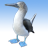# Calculate Circular Mean Statistics Option

452
0
12-02-2019 09:31 AM
Status: Open
Labels (1)New Contributor III

It would be nice if the mean of circular data could be calculated using the basic table statistics function.  For example, when aspect values are extracted to point locations, the arithmetic mean is useless because the data are circular (e.g., azimuths 1 and 359 are both nearly due north, but their arithmetic average is 180...due south).  It can be very frustrating trying to understand/explain the correct formula to calculate the "average" slope direction to students or less-than-savvy GIS users and I suspect many reports have included erroneous results.

A simple option to calculate circular mean in the 'Data' tab of the chart properties in ArcGIS Pro after clicking statistics for an attribute table field would be great.

Tags (4)